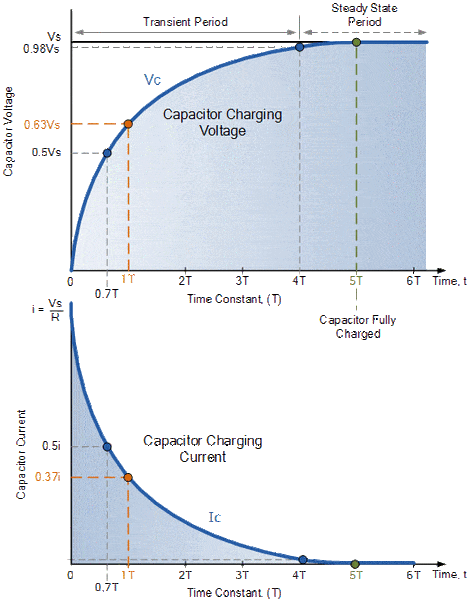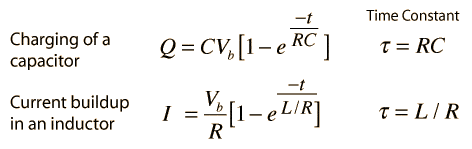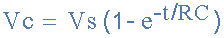# How many electrons on a Capacitor calculation

Hello,
How to find number of electronics in capacitor?
as 1 coulomb and 1 Amp has 6.25x10^18
on what they depend?
Is this correct..?
Q=C*V
C=47uF and V=12V
Q=47*10^-6*12=5.64*10^-4 Coulmb?????

Drakkith
Staff Emeritus
How to find number of electronics in capacitor?

The number of electrons in a capacitor is given by Q=CV, which you've calculated correctly.

Thanks.

I don't understand this graph
How the capacitor will charge with series Resistance?Drakkith
Staff Emeritus
•diogenesNY and ENE
Hello,
I got
a. The rate of charging is typically described in terms of a time constant RC.
b. The electrical transient phenomena in capacitors and inductors are exponential processesvoltage across the capacitor
how we get exponential here?The voltage on a capacitor is Vc = Q / C.

Note: you copied images from two different circuit explanations and that's causing confusion. The "Vb" in the first image is "Vs" in the second image.

•ENE
i have to learn this equation?

Staff Emeritus
2021 Award
i have to learn this equation?

Oh, the horror! The horror!

Yes, you have to learn it.

•ProfuselyQuarky
in electrical engineering how man equation are there?

jtbell
Mentor
voltage across the capacitor
how we get exponential here?We solve the differential equation for the circuit. See for example

http://web.mit.edu/molly/Public/circuits-b.pdf

and scroll down to page W6-6. This uses Q as the variable, not V, but you can change variables using Q = CV if you prefer.

Hello,
Why The sum of the voltage drops∆Vi , across any circuit elements that form a closed circuit is zero.??

ProfuselyQuarky
Gold Member
The voltage on a capacitor is Vc = Q / C.
Oh, the horror! The horror!

Yes, you have to learn it.
ENE, that's an awfully basic equation, you needn't worryDrakkith
Staff Emeritus
Hello,
Why The sum of the voltage drops∆Vi , across any circuit elements that form a closed circuit is zero.??

The answer to this is kind of in-depth and I don't feel you're ready for it at the moment.

Hello,
Why The sum of the voltage drops∆Vi , across any circuit elements that form a closed circuit is zero.??
The static electric field is a conservative field. So the work done on a closed path is zero.
Do you understand the relationship between work and potential difference?